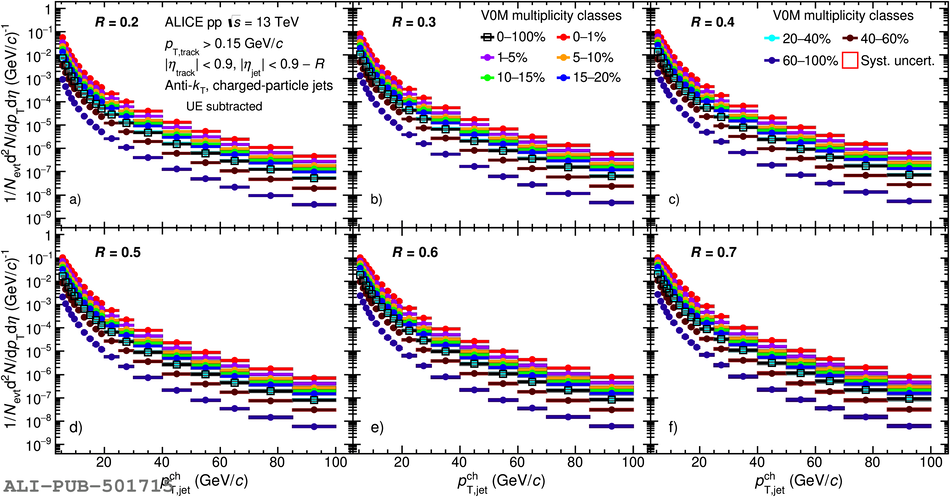# Figure 7

 Charged-particle jet yields in different V0M multiplicity percentile intervals for resolution parameters $R$ varied from $0.2$ to $0.7$ in pp collisions at $\sqrt{s} = 13\ \mathrm{TeV}$. Statistical and total systematic uncertainties are shown as vertical error bars and boxes around the data points, respectively.